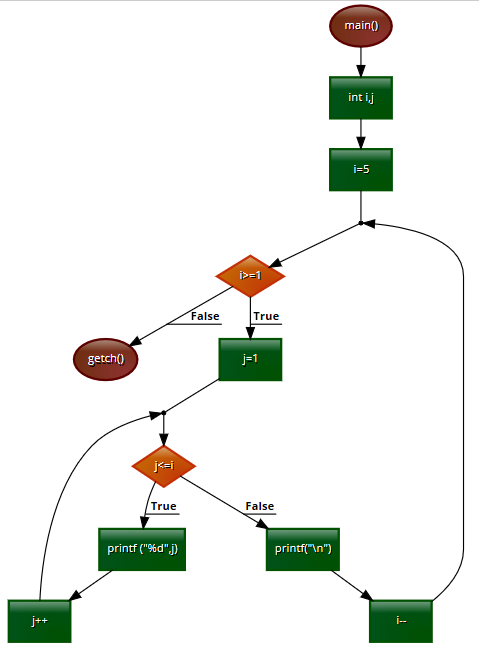# Pattern printing 1 :- using FOR LOOP - C Programming Example

1 2 3 4 5

1 2 3 4
1 2 3
1 2
1

This program is an example of pattern printing by using the for loop in the programming language C.

## C Programming Code

#include<stdio.h>
#include<conio.h>
main()
{
int i,j;
for(i=5;i>=1;i--)
{
for(j=1;j<=i;j++)
{
printf ("%d",j);
}
printf("\n");
}
getch();
}

## Output of the codeNumber Pattern 1

## Explanation of the code

·        #include<stdio.h>

#include<stdio.h>
Ø  This are called header file. A header file is a file with extension .h which contains C function declarations and macro definitions to be shared between several source files The C programming language provides many standard library functions for file input and output. These functions make up the bulk of the C standard library header <stdio.h>.In the other hand  <conio.h> is a C header file used mostly by MS-DOS compilers to provide console input/output. It is not part of the C standard library or ISO C. This header declares several useful library functions for performing "console input and output" from a program.

·         main()
Ø  In C, program execution starts from the main() function. The main function can in-turn call other functions. When main calls a function, it passes the execution control to that function. The function returns control to main when a return statement is executed or when end of function is reached.
int i,j;
int is a variable or data type.  A variable declared to be of type int can be used for storing numbers which do not have decimal points. Here we declare “I,j” variable in int type.
for(i=5;i>=1;i--)
{
for(j=1;j<=i;j++)
As the problem has to be solved by usong for loop that’s why for loop is being used in this program. Here I is used as row indicator and j is used as column indicator.
for(i=5;i>=1;i--)
The condition for the loop first loop is quite simple. As the pattern is

12345
1234
123
12
1
Or in other words starts from one and gradually increases but in each line the one number is being deducted when printed. That deduction will be explained in the next loop. Here as I is the row indicator the contents of  the row are being explained in this loop. At first the loop will have 5 numbers so the first condition is i=5
Then the contents of the rows will keep running till I becomes greater or equal 1. The question may arise why the loop wont end after just printing 1. Or why is it ending on the last line.
These questions will be answered in the next loop too.
The last condition of this loop is i—or in other words I will gradually decrease. Hence it will stop at 1 , right at the last line.
for(j=1;j<=i;j++)
Now coming to the column printing, as always the column will start from 1 and keep on running until it becomes less or equal then i. If i=5, 5 columns will print, if i=4, 4 columns will print and so on. And finally the contents of the column will keep increasing as the last condition for the loop is j ++
Lets see this now code through a flow chartFlow chart of the c programming code

And finally getch(); is written to end the program.